# The Duocylinder

## Construction

We have seen that the prismic cylinders are formed by taking the limit of the m,n-duoprisms as m approaches infinity. We need not stop with the prismic cylinders; we can now take the limit of n-gonal prismic cylinders as n approaches infinity.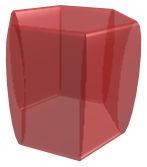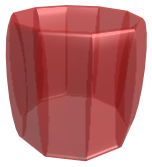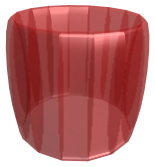As n increases, so does the number of constituent cylinders; but their height also decreases to zero. The number of faces in the ZW torus also increase without bound, and it becomes increasingly close to being circular. At the limiting case, the ring of cylinders becomes a circular torus, and the ZW polygonal torus also becomes a circular torus. These two tori are in fact identical (although they appear different in the projections we see here).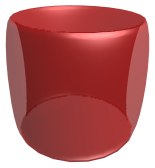This resulting object is called a duocylinder. It is the 4D volume described by the equations:

x2 + y2 ≤ r2
z2 + w2 ≤ r2

It is bounded by two equivalent, mutually perpendicular, circular tori, described respectively by the equations:

x2 + y2 ≤ r2, z2 + w2 = r2;
z2 + w2 ≤ r2, x2 + y2 = r2

The following animation shows the duocylinder rotating in the YW plane. The red part represents half of the duocylinder, and the green part the other half.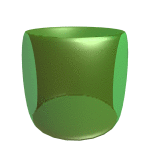## Properties

The duocylinder is a peculiar object. Its two torus-shaped boundaries are surfaces that it can roll on, like a wheel. They are mutually perpendicular, so when rolling on one side, the duocylinder can only cover the space of a line. But if you tip it sideways on the other side, it will roll along a perpendicular line. It can always roll no matter which side you stand it on, but in different perpendicular directions depending on which side it is standing on.

Last updated 02 Feb 2023.